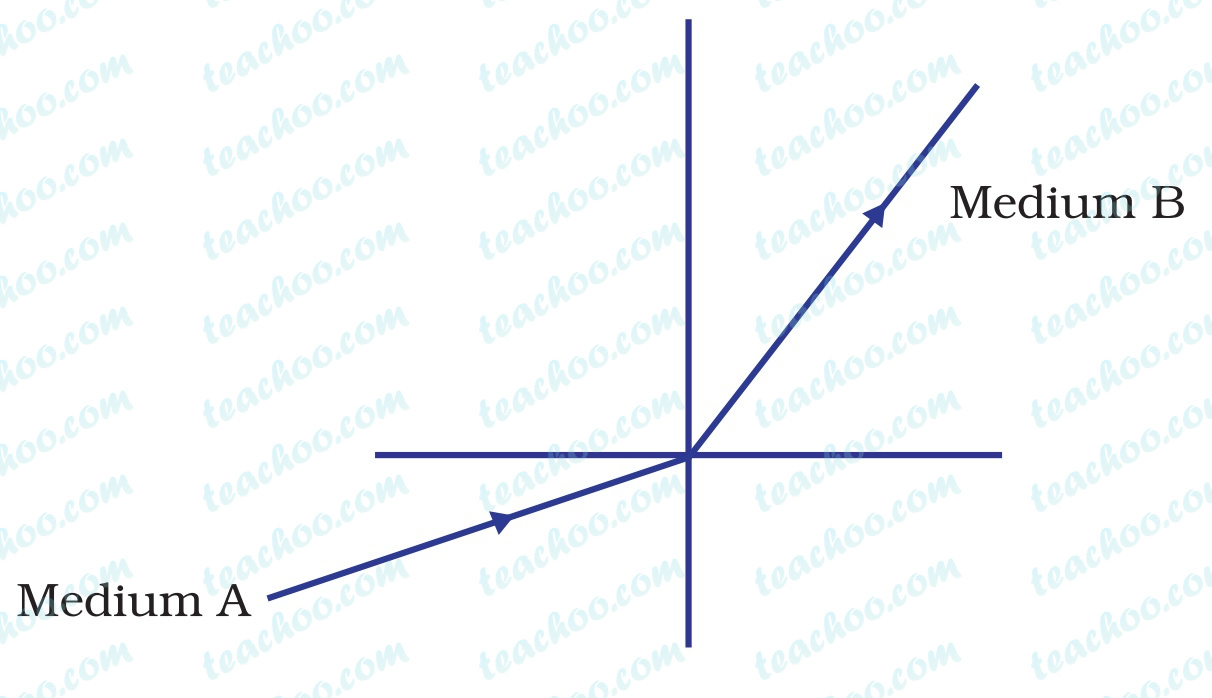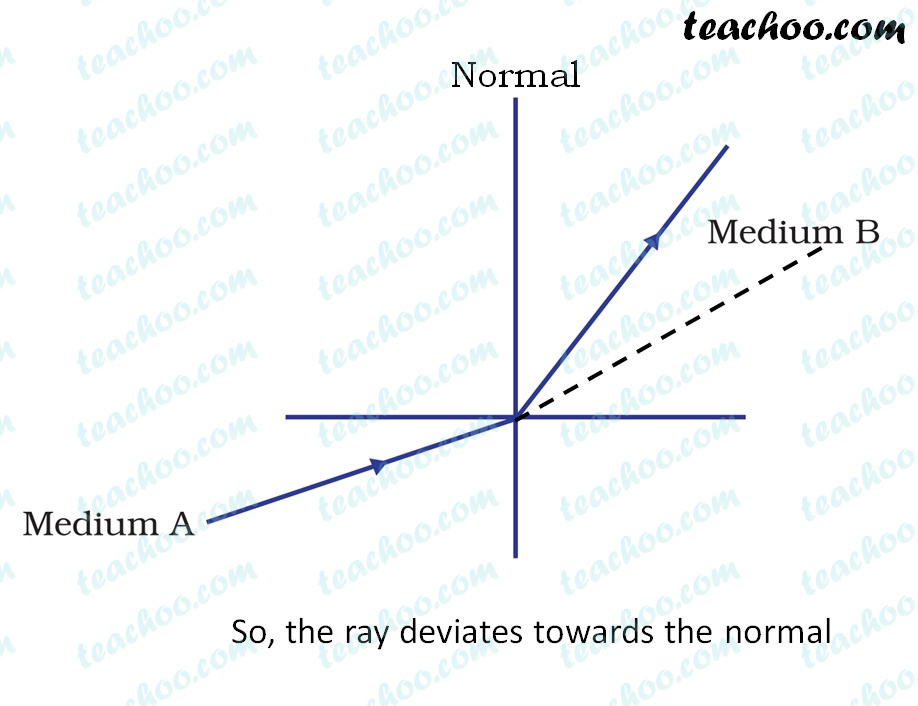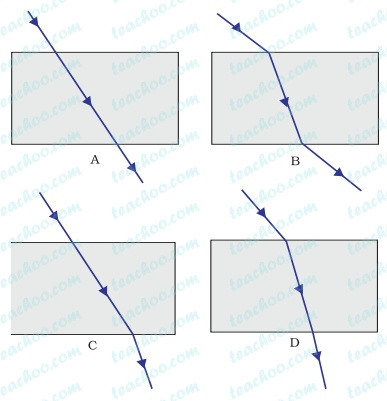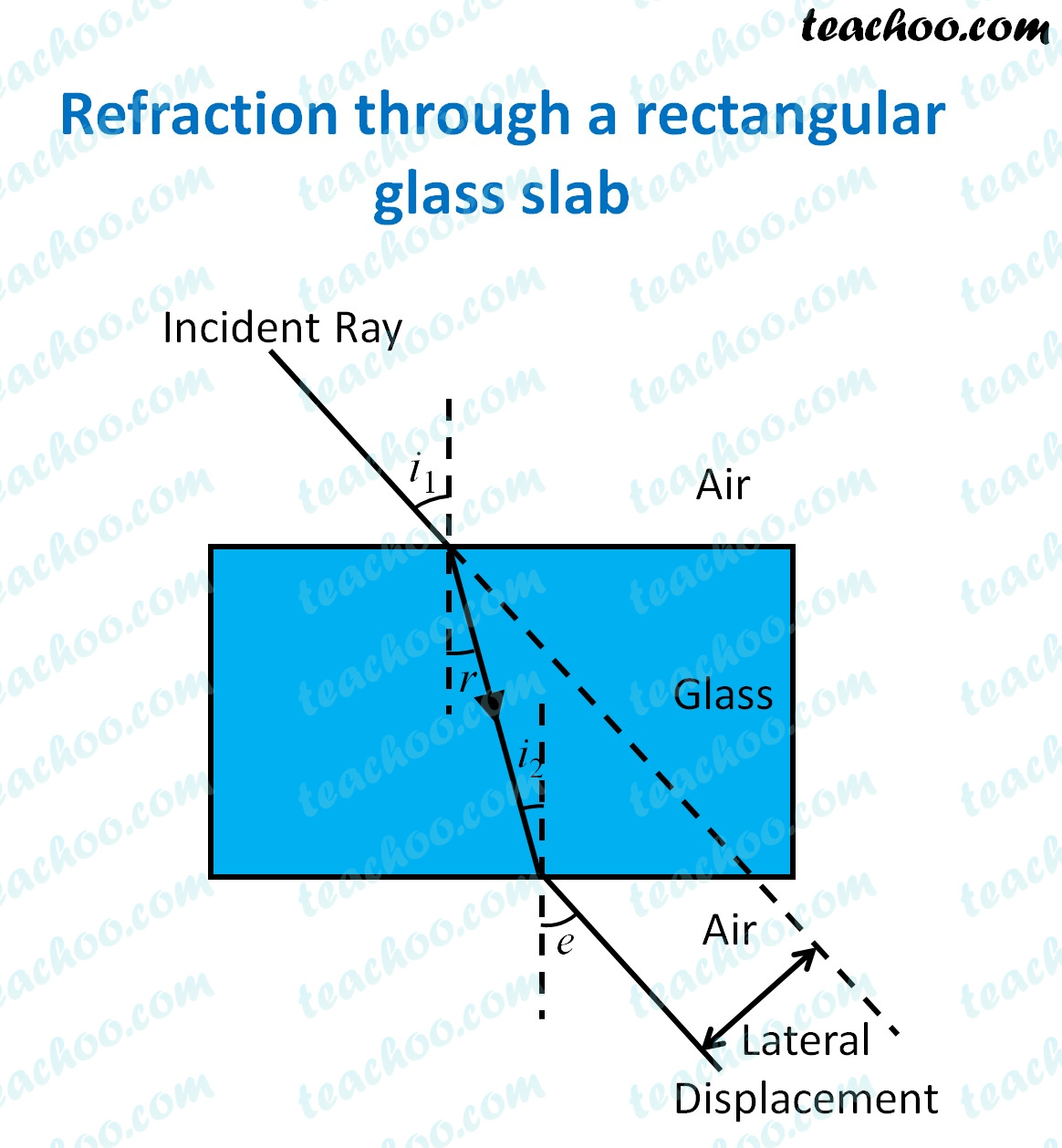Teachoo Questions

Class 10
Chapter 10 Class 10 - Light - Reflection and Refraction

The password to open the file is -    teachooisbest

## A light ray enters from medium A to medium B as shown in Figure 10 The refractive index of medium B relative to A will be1. greater than unity
2. less than unity
3. equal to unity
4. zero

greater than unity

Explanation

###We see that

Ray of light bends towards the normal as we go from medium A to medium B

And we know that

When ray goes from rarer to denser medium, it bends towards normal

So, that means Refractive index of B is greater than A

Thus, Refractive index of B relative than A = Refractive index of B/Refractive Index of A

Since,  Refractive index of B > Refractive index of A

Therefore,

Refractive index of B relative than A > 1

## Which of the following statements is true?

1.  A convex lens has 4 dioptre power having a focal length 0.25 m
2. A convex lens has –4 dioptre power having a focal length 0.25 m
3. A concave lens has 4 dioptre power having a focal length 0.25 m
4. A concave lens has –4 dioptre power having a focal length 0.25 m

(a) A convex lens has 4 dioptre power having a focal length 0.25 m

Explanation

In all the cases,

Focus length is positive

So, it will be a convex lens

Now,

Power of a lens = 1/ focal length

For focal length = 0.25m

Power of the lens = 1/0.25

= 100/25

= 4 D

Thus, it is a convex lens with power 4 D

So, (a) is the correct answer

## The laws of reflection hold good for

1. plane mirror only
2. concave mirror only
3. convex mirror only
4. all mirrors irrespective of their shape

all mirrors irrespective of their shape

Explanation -  The laws of reflection are obeyed by all mirrors irrespective of their shape surface.

## The path of a ray of light coming from air passing through a rectangular glass slab traced by four students are shown as A, B, C and D in Figure. Which one of them is correct?1. D

(b) B

Explanation - The correct image looks likeThe refractive index of glass is more than the refractive index of air.

Hence, glass is more optically dense than air.

Case I - Ray of light enters from air to glass

When a ray of light enters from air to glass, it bends towards the normal.

Case II - Ray of light enters from glass to air

When a ray of light enters from glass to air, it bends away from the normal.

The above two cases are only depicted correctly in (B),

Hence (B) is the correct answer

## A child is standing in front of a magic mirror. She finds the image of her head bigger, the middle portion of her body of the same size and that of the legs smaller. The following is the order of combinations for the magic mirror from the top.

1. Plane, convex and concave
2. Convex, concave and plane
3. Concave, plane and convex
4. Convex, plane and concave

Concave, plane and convex

Explanation - The image of the head is larger. An enlarged image is formed by a concave mirror, hence the mirror on the top is a concave mirror .

The middle portion of the body is of the same size.

An image of the same size is formed by a plane mirror, hence the middle part of the mirror is a plane mirror .

The image of the legs is smaller.

A diminished image is formed by a convex mirror, hence the mirror on the bottom is a convex mirror .

Get live Maths 1-on-1 Classs - Class 6 to 12